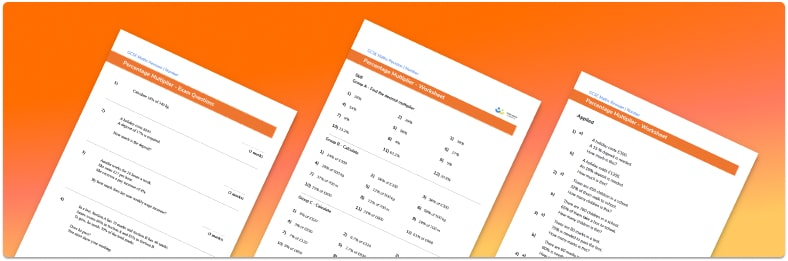# Percentage Multiplier Worksheet• Section 1 of the percentage multiplier worksheet contains 36 skills-based percentage multiplier questions, in 3 groups to support differentiation
• Section 2 contains 4 applied percentage multiplier questions with a mix of worded problems and deeper problem solving questions
• Section 3 contains 4 foundation and higher level GCSE percentage multiplier exam questions
• Answers and a mark scheme for all percentage multiplier questions are provided
• Questions follow variation theory with plenty of opportunities for students to work independently at their own level
• All questions created by fully qualified expert secondary maths teachers

Suitable for GCSE maths revision for AQA, OCR and Edexcel exam boards

• This field is for validation purposes and should be left unchanged.

You can unsubscribe at any time (each email we send will contain an easy way to unsubscribe). To find out more about how we use your data, see our privacy policy.

### Percentage multiplier at a glance

A percentage multiplier is the decimal equivalent of a percentage, it is used to calculate percentages of amounts, percentage increase and percentage decrease.

When finding percentages of amounts, there are some quantities that students are expected to calculate mentally – such as 50%, 25%, 10%. For trickier amounts (e.g. 47% of 320), the decimal multiplier method is more efficient. We use the decimal equivalent of 47%, which is 0.47, and rewrite the calculation as 0.47 320.

When calculating percentage increase, we take account of the fact that we’re adding to the original amount (100%), so the percentage multiplier for an increase of 7% would be 1.07. When calculating percentage decrease, we’re subtracting from 100%, so the multiplier for a decrease of 12% would be 0.88 (1 0.12). This skill has lots of real-life applications, such as calculating sale prices. Repeated percentage change links to ideas such as interest rates and compound depreciation.

Looking forward, students can then progress to additional number worksheets, for example a standard form worksheet or a.For more teaching and learning support on Number our GCSE maths lessons provide step by step support for all GCSE maths concepts.

## Do you have KS4 students who need more focused attention to succeed at GCSE?There will be students in your class who require individual attention to help them succeed in their maths GCSEs. In a class of 30, it’s not always easy to provide.

Help your students feel confident with exam-style questions and the strategies they’ll need to answer them correctly with our dedicated GCSE maths revision programme.

Lessons are selected to provide support where each student needs it most, and specially-trained GCSE maths tutors adapt the pitch and pace of each lesson. This ensures a personalised revision programme that raises grades and boosts confidence.

Find out more